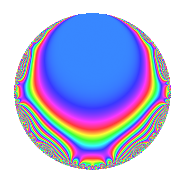# Properties

 Label 630.2.d.aLevel 630 Weight 2 Character orbit 630.d Analytic conductor 5.031 Analytic rank 0 Dimension 4 CM No Inner twists 4

# Related objects

## Newspace parameters

 Level: $$N$$ = $$630 = 2 \cdot 3^{2} \cdot 5 \cdot 7$$ Weight: $$k$$ = $$2$$ Character orbit: $$[\chi]$$ = 630.d (of order $$2$$ and degree $$1$$)

## Newform invariants

 Self dual: No Analytic conductor: $$5.03057532734$$ Analytic rank: $$0$$ Dimension: $$4$$ Coefficient field: $$\Q(\sqrt{-2}, \sqrt{-5})$$ Coefficient ring: $$\Z[a_1, \ldots, a_{11}]$$ Coefficient ring index: $$1$$ Sato-Tate group: $\mathrm{SU}(2)[C_{2}]$

## $q$-expansion

Coefficients of the $$q$$-expansion are expressed in terms of a basis $$1,\beta_1,\beta_2,\beta_3$$ for the coefficient ring described below. We also show the integral $$q$$-expansion of the trace form.

 $$f(q)$$ $$=$$ $$q - q^{2} + q^{4} + \beta_{3} q^{5} + ( -\beta_{1} - \beta_{2} ) q^{7} - q^{8} +O(q^{10})$$ $$q - q^{2} + q^{4} + \beta_{3} q^{5} + ( -\beta_{1} - \beta_{2} ) q^{7} - q^{8} -\beta_{3} q^{10} + \beta_{2} q^{11} + ( -2 \beta_{1} + \beta_{2} ) q^{13} + ( \beta_{1} + \beta_{2} ) q^{14} + q^{16} + 2 \beta_{3} q^{17} + \beta_{3} q^{20} -\beta_{2} q^{22} -6 q^{23} -5 q^{25} + ( 2 \beta_{1} - \beta_{2} ) q^{26} + ( -\beta_{1} - \beta_{2} ) q^{28} -2 \beta_{2} q^{29} - q^{32} -2 \beta_{3} q^{34} + ( 3 \beta_{1} - 4 \beta_{2} ) q^{35} + 3 \beta_{2} q^{37} -\beta_{3} q^{40} + ( -6 \beta_{1} + 3 \beta_{2} ) q^{41} -6 \beta_{2} q^{43} + \beta_{2} q^{44} + 6 q^{46} + 2 \beta_{3} q^{47} + ( -2 + 3 \beta_{3} ) q^{49} + 5 q^{50} + ( -2 \beta_{1} + \beta_{2} ) q^{52} -6 q^{53} + ( -2 \beta_{1} + \beta_{2} ) q^{55} + ( \beta_{1} + \beta_{2} ) q^{56} + 2 \beta_{2} q^{58} + ( -6 \beta_{1} + 3 \beta_{2} ) q^{59} + 6 \beta_{3} q^{61} + q^{64} -5 \beta_{2} q^{65} + 2 \beta_{3} q^{68} + ( -3 \beta_{1} + 4 \beta_{2} ) q^{70} + 4 \beta_{2} q^{71} + ( 4 \beta_{1} - 2 \beta_{2} ) q^{73} -3 \beta_{2} q^{74} + ( 3 - \beta_{3} ) q^{77} -4 q^{79} + \beta_{3} q^{80} + ( 6 \beta_{1} - 3 \beta_{2} ) q^{82} -4 \beta_{3} q^{83} -10 q^{85} + 6 \beta_{2} q^{86} -\beta_{2} q^{88} + ( 6 \beta_{1} - 3 \beta_{2} ) q^{89} + ( 5 + 3 \beta_{3} ) q^{91} -6 q^{92} -2 \beta_{3} q^{94} + ( -8 \beta_{1} + 4 \beta_{2} ) q^{97} + ( 2 - 3 \beta_{3} ) q^{98} +O(q^{100})$$ $$\operatorname{Tr}(f)(q)$$ $$=$$ $$4q - 4q^{2} + 4q^{4} - 4q^{8} + O(q^{10})$$ $$4q - 4q^{2} + 4q^{4} - 4q^{8} + 4q^{16} - 24q^{23} - 20q^{25} - 4q^{32} + 24q^{46} - 8q^{49} + 20q^{50} - 24q^{53} + 4q^{64} + 12q^{77} - 16q^{79} - 40q^{85} + 20q^{91} - 24q^{92} + 8q^{98} + O(q^{100})$$

Basis of coefficient ring in terms of a root $$\nu$$ of $$x^{4} - 4 x^{2} + 9$$:

 $$\beta_{0}$$ $$=$$ $$1$$ $$\beta_{1}$$ $$=$$ $$\nu$$ $$\beta_{2}$$ $$=$$ $$($$$$\nu^{3} - \nu$$$$)/3$$ $$\beta_{3}$$ $$=$$ $$\nu^{2} - 2$$
 $$1$$ $$=$$ $$\beta_0$$ $$\nu$$ $$=$$ $$\beta_{1}$$ $$\nu^{2}$$ $$=$$ $$\beta_{3} + 2$$ $$\nu^{3}$$ $$=$$ $$3 \beta_{2} + \beta_{1}$$

## Character Values

We give the values of $$\chi$$ on generators for $$\left(\mathbb{Z}/630\mathbb{Z}\right)^\times$$.

 $$n$$ $$127$$ $$281$$ $$451$$ $$\chi(n)$$ $$-1$$ $$-1$$ $$-1$$

## Embeddings

For each embedding $$\iota_m$$ of the coefficient field, the values $$\iota_m(a_n)$$ are shown below.

For more information on an embedded modular form you can click on its label.

Label $$\iota_m(\nu)$$ $$a_{2}$$ $$a_{3}$$ $$a_{4}$$ $$a_{5}$$ $$a_{6}$$ $$a_{7}$$ $$a_{8}$$ $$a_{9}$$ $$a_{10}$$
629.1
 1.58114 − 0.707107i −1.58114 + 0.707107i 1.58114 + 0.707107i −1.58114 − 0.707107i
−1.00000 0 1.00000 2.23607i 0 −1.58114 + 2.12132i −1.00000 0 2.23607i
629.2 −1.00000 0 1.00000 2.23607i 0 1.58114 2.12132i −1.00000 0 2.23607i
629.3 −1.00000 0 1.00000 2.23607i 0 −1.58114 2.12132i −1.00000 0 2.23607i
629.4 −1.00000 0 1.00000 2.23607i 0 1.58114 + 2.12132i −1.00000 0 2.23607i
 $$n$$: e.g. 2-40 or 990-1000 Significant digits: Format: Complex embeddings Normalized embeddings Satake parameters Satake angles

## Inner twists

Char. orbit Parity Mult. Self Twist Proved
1.a Even 1 trivial yes
7.b Odd 1 yes
15.d Odd 1 yes
105.g Even 1 yes

## Hecke kernels

This newform can be constructed as the intersection of the kernels of the following linear operators acting on $$S_{2}^{\mathrm{new}}(630, [\chi])$$:

 $$T_{11}^{2} + 2$$ $$T_{23} + 6$$